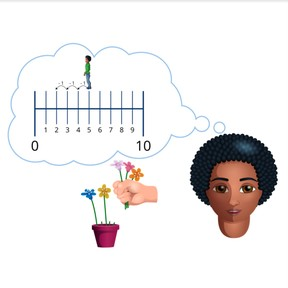Subtraction to 10 on the number line

# Subtraction to 10 on the number line8,000 schools use Gynzy92,000 teachers use Gynzy1,600,000 students use Gynzy

## General

Students learn subtraction with numbers to 10 on the number line.

1.OA.A
1.OA.C.5,
1.OA.C.6

## Relevance

It is important to be able to subtract, so you can determine how many you have left after taking some away. Being able to use the number line helps make subtraction easier.

## Introduction

The interactive whiteboard shows three number lines with missing numbers. Students must drag the missing numbers to their places on the number line.

## Development

Start by showing students that it is possible to subtract using a number line. Show them how to subtract on a number line using three steps. First students must look at the first number (minuend) of the subtraction problem and move to that number on the number line. Secondly, students must look at the second number (subtrahend) of the subtraction problem to determine how many steps they must jump back on the number line. The third step is to determine which number they end at, which is their difference. To check that students can subtract using the number line, ask students to complete the subtraction problems given on the interactive whiteboard as a class. Remind students that they can choose how big to make their jumps back on the number line (by one, two, etc).

To check that students can subtract with numbers to 10 on the number line, you can ask the following questions:
- Which number do you look at to determine where you start on the number line?
- Which number do you look at to determine how many jumps back you need to take?
- How do you know what the answer is?

## Guided practice

Students practice subtraction on the number line, with increasingly larger numbers (subtrahends).

## Closing

Ask ten students to come to the front of the class and represent a living number line. Give them number cards from 0-10. Name a subtraction problem. Ask one student to come to the front of the class. The rest of the class must place that student "on" the number line and instruct them on how many jumps to take to solve the problem. Ask them how they know where to place the student and how they determine how many jumps they must take. Repeat with a different subtraction problem and new student at the front of the class to move "on" the number line.

## Teaching tips

Students who have difficulty with this goal can be supported by extra revision of the number line to 10. They can write all the intermediate numbers between 1 and 10 on the number line. Have them practice with taking one step back at a time.

## Instruction materials

Number cards with the numbers 0-10.

### The online teaching platform for interactive whiteboards and displays in schools

• Save time building lessons

• Manage the classroom more efficiently

• Increase student engagement